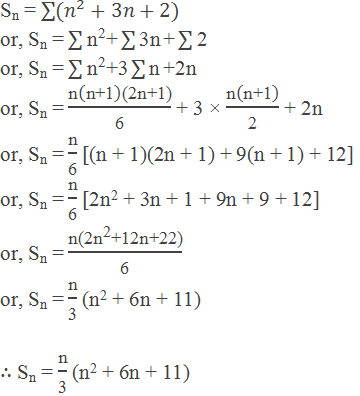# Sum of Arithmetic Series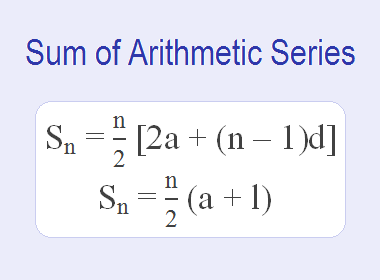## Arithmetic Series

The series associated with an arithmetic sequence is known as an arithmetic series. For example: 3 + 7 + 11 + 15 + 19 + 23 + 27 + 31 is the arithmetic series associated with the arithmetic sequence: 3, 7, 11, 15, 19, 23, 27, 31.

## Sum of Arithmetic Series

To derive the formula for the sum of arithmetic series, let us suppose an arithmetic series where a be the first term, d be the common difference, n be the number of terms, l be the last term and Sn be the sum of the n terms of an arithmetic series (AS), then,

Sn = a + (a + d) + (a + 2d) + … … … + (l – 2d) + (l – d) + l ………… (i)

Writing in the reverse order, we have,

Sn = l + (l – d) + (l – 2d) + … … … + (a + 2d) + (a + d) + a ………… (ii)

Adding relations (i) and (ii), we get

2Sn = (a + l) + (a + l) + (a + l) + … … … + (a + l) + (a + l) + (a + l).

or, 2Sn = n(a + l)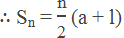Again, the last term, l = a + (n – 1)d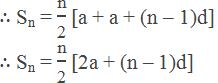Therefore, the sum of the arithmetic series can be found by using the following formulas: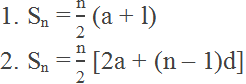### Worked Out Examples

Example 1: Find the sum of the series 2 + 8 + 14 + 20 + … … … 20 terms.

Solution:

Here,

First term (a) = 2

Common difference (d) = 8 – 2 = 6

Number of terms (n) = 20

Sum (Sn) = ?

We Know,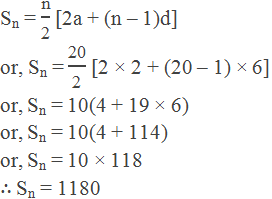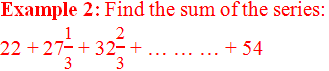Solution:

Here,

First term (a) = 22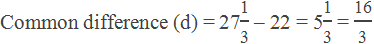Last term (l) = 54

Number of terms (n) = ?

Sum (Sn) = ?

We know,

l = a + (n – 1)d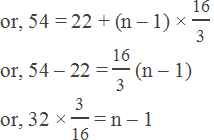or, n – 1 = 6

n = 7

Now,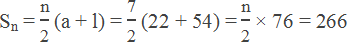Hence, the required sum is 266.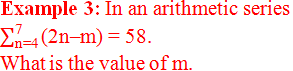Solution:

Given,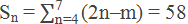or, (3×4 – m)+ (3×5 – m)+ (3×6 – m)+ (3×7 – m) = 58

or, (12 – m)+ (15 – m)+ (18 – m)+ (21 – m) = 58

or, 12 – m + 15 – m + 18 – m + 21 – m = 58

or, 66 – 4m = 58

or, 4m = 66 – 58

or, 4m = 8

m = 2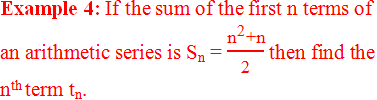Solution:

Given,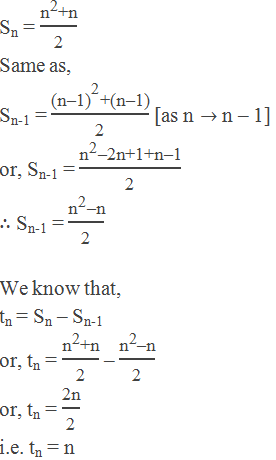Example 5: How many terms of an AS 17 + 15 + 13 + … … are needed to give the sum 80?

Solution:

Here,

First term (a) = 17

Common difference (d) = -2

Sum (Sn) = 80

Number of terms (n) = ?

By using formula,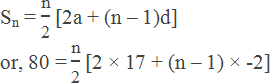or, 160 = n[34 – 2n + 2]

or, 2n2 – 36n + 160 = 0

or, n2 – 18n + 80 = 0

or, n2 – 10n – 8n + 80 = 0

or, (n – 10)(n – 8) = 0

n = 8 or 10

Example 6: If the sum of the first 16 terms of an AS is 280 and the 6th term of the series is 14. Find the sum of the first 21 terms.

Solution:

Let a be the first term and d be the common difference of the given series.

Sum of 16 terms (S16) = 280

6th term (t6) = 14

By using formula, we have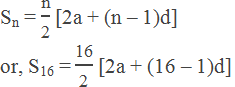or, 280 = 8(2a + 15d)

or, 2a + 15d = 35 …………… (i)

Again,

tn = a + (n – 1)d

or, t4 = a + (6 – 1)d

or, a + 5d = 14 …………… (ii)

Solving equations (i) and (ii), we get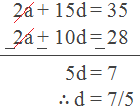Substituting the value in equation (ii),

a + 5 × 7/5 = 14

or, a + 7 = 14

or, a = 7

Now,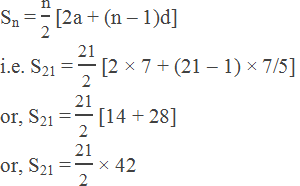or, S21 = 441

Hence, the sum of 21 terms of the series is 441.

Example 7: Find the sum of all natural numbers less than 100 which are exactly divisible by 7.

Solution:

Here,

First term (a) = 7

Common difference (d) = 7

Last term (l) =98

Sum (Sn) = ?

Number of terms (n) = ?

By using formula,

l = a + (n – 1)d

or, 98 = 7 + (n – 1)7

or, 98 = 7 + 7n – 7

or, 7n = 98

or, n = 14

Hence by using formula,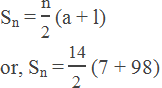or, S14 = 7 × 105

S14 = 735

The required sum is 735.

## Sum of the First n Natural Numbers

The first n natural numbers are 1, 2, 3, 4, … … … n.

Let Sn = 1 + 2 + 3 … … … + n

Here,

First term (a) = 1

Common difference (d) = 2 – 1 = 1

Number of terms (n) = n

Sum of first n terms (Sn) = ?

By using formula,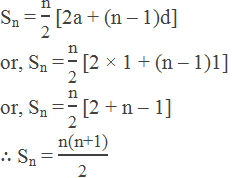## Sum of the First n Odd Natural Numbers

The first n odd natural number are 1, 3, 5, … … …, (2n – 1)

Let Sn = 1 + 3 + 5 + … … + (2n – 1)

Here,

First term (a) = 1

Common difference (d) = 3 – 1 = 2

Number of terms (n) = n

Sum of n terms (Sn) = ?

By using formula,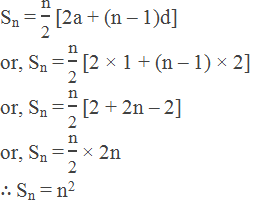## The Sum of the First n Even Natural Numbers

The first n even natural numbers are 2, 4, 6, … …, 2n.

Let Sn = 2 + 4 + 6 + … … + 2n

Here,

First term (a) = 2

Common difference (d) = 4 – 2 = 2

Number of terms (n) = n

Sum of n terms (Sn) = ?

By using formula,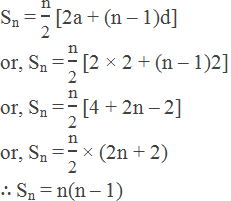## Sum of the Squares of the First n Natural Numbers

The squares of the first n natural numbers are 12, 22, 32, … …, n2

Let Sn = 12 + 22 + 32 + … … + n2

Consider the identity,

r3 – (r – 1)3 = r3 – (r3 – 3r2 + 3r – 1)

or, r3 – (r – 1)3 = 3r2 – 3r + 1

Putting r = 1, 2, 3, 4, … …, (n – 1), n

13 – 03 = 3.12 – 3.1 + 1

23 – 13 = 3.22 – 3.2 + 1

33 – 23 = 3.32 – 3.3 + 1

43 – 33 = 3.42 – 3.4 + 1

… … … … … … … …

(n – 1)3 – (n – 2)3 = 3(n – 1)2 – 3(n – 1) + 1

n3 – (n – 1)3 = 3n2 – 3n + 1

Adding above all identities, we have

n3 = 3(12 + 22 + 32 + … … + n2) – 3(1 + 2 + 3 + … … + n) + n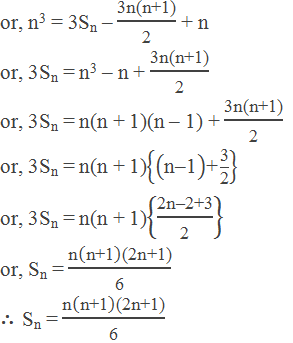## Sum of the Cubes of the First n Natural Numbers

The cubes of the first n natural numbers are 13, 23, 33, … …, n3.

Let Sn = 13 + 23 + 33 + … … + n3

Consider the identity,

k4 – (k – 1)4 = k4 – (k4 – 4k3 + 6k2 – 4k + 1)

or, k4 – (k – 1)4 = 4k3 – 6k2 + 4k – 1

Putting k = 1, 2, 3, 4, … …, (n – 1), n.

14 – 04 = 4.13 – 6.12 + 4.1 – 1

24 – 14 = 4.23 – 6.22 + 4.2 – 1

34 – 24 = 4.33 – 6.32 + 4.3 – 1

44 – 34 = 4.43 – 6.42 + 4.4 – 1

… … … … … … … … … …

(n – 1)4 – (n – 2)4 = 4.(n – 1)3 – 6.(n – 1)2 + 4.(n – 1) – 1

n4 – (n – 1)4 = 4.n3 – 6.n2 + 4.n – 1

Adding all above identities, we have,

n4 = 4(13 + 23 + 33 + … … + n3) – 6(12 + 22 + 32 + … … + n2) + 4(1 + 2 + 3 + … … + n) – n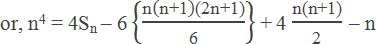or, 4Sn = n4 + n + n(n + 1)(2n + 1) – 2n(n + 1)

or, 4Sn = n(n + 1)(n2 – n + 1) + n(n + 1)(2n + 1) – 2n(n + 1)

or, 4Sn = n(n + 1)(n2 – n + 1 + 2n + 1 – 2)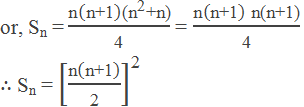### More Examples

Example 8: Find the sum of the following series:

a. 2 + 4 + 6 + … … … 12 terms

b. 13 + 23 + 33 + … … 10 terms

Solution:

a. The given series is 2 + 4 + 6 + … … … 12 terms

This is the sum of the first 12 even natural numbers.

Here, n = 12

Sn = n(n + 1) = 12(12 + 1) = 156

b. The given series is 13 + 23 + 33 + … … 10 terms

This is the sum of the cubes of the first 10 natural numbers

Here, n = 10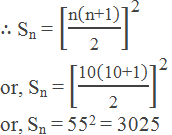Example 9: The sum of three numbers in AP is 27 and the sum of their squares is 293. Find the numbers.

Solution:

Let the three numbers in AP be a – d, a and a + d.

Then,

(a – d) + a + (a + d) = 27

i.e. a = 9

And,

(a – d)2 + a2 + (a + d)2 = 293

or, a2 – 2ad + d2 + a2 + a2 + 2ad + d2 = 293

or, 3a2 + 2d2 = 293

or, 3 × 92 + 2d2 = 293

or, d2 = 25

or, d = 5

Hence, the required numbers are a – d, a and a + d.

i.e. 4, 9 and 14.

Example 10: Find nth term and the sum of n terms of the series 2×3 + 3×4 + 4×5 + … … n terms.

Solution:

The given series is 2×3 + 3×4 + 4×5 + … … n terms.

nth term of 2, 3, 4, … … = 2 + (n – 1)1 = n + 1

nth term of 3, 4, 5, … … = 3 + (n – 1)1 = n + 2

The nth term of the given series = (n + 1)(n + 2) = n2 + 3n + 2

Sum of the series of n terms,